《密码协议》课程笔记（1） 引论+密钥交换协议

## 引论

### 密码协议基础

• 协议：两个或者两个以上的参与者为了达到特定的目的，采取的一系列步骤（没有严格的界限来区分密码协议和一般协议）
• 规定了一系列有序执行的步骤
• 有两个或者两个以上的参与者
• 协议都有明确的目的
• 密码协议的要求：
• 安全需求——加密（执行过程中的敏感数据不被非授权者所知）、认证（保证参与者的合法身份）和不可抵赖性（协议执行过程可以追查）
• 鲁棒性——有恶意参与者时能部分地正确执行
• 分类：
• 仲裁协议——仲裁者是可信第三方，协议的执行一直需要仲裁者
• 裁决协议——裁决者只在有争议的时候才参与协议的执行
• 自动执行协议——协议本身公平，不需要可信第三方

### 密码协议模型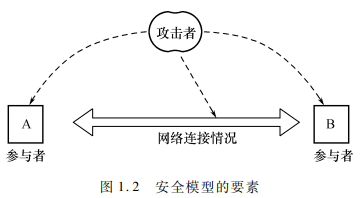• 参与密码协议的主体：协议参与者、协议攻击者、可信第三方（协议参与者都信任的一个主体或者一个组织）、仲裁者——攻击者和参与者没有严格的界限
• 网络可能是同步的，也可能是非同步的。同步网络中，所有信息在某一个时钟周期内传送。大部分密码协议的研究都在同步网络条件下
• 参与者分为诚实参与者、半诚实参与者和恶意攻击者
• 攻击者的能力：拥有无限计算能力的攻击者，只有概念多项式时间计算能力的攻击者——信息论安全的、密码学安全的
• 安全信道：参与者的通信既不会被攻击者窃听，也不会被攻击者篡改
• 认证的信道：攻击者可以窃听参与者的通信，但是不能篡改通信内容
• 未认证的信道：窃听+篡改
• 被动攻击者（窃听者）：只是窃听或获取不诚实参与者在协议进行中的所有输入，不控制其行为
• 主动攻击者：窃听+控制行为

### 密码协议设计分析概述

• 密码协议安全性分析的基本方法：
• 使用自然语言论证的标准模型
• 开发专家系统
• 使用Random Oracle模型
• 使用BAN类逻辑
• 使用CSP（Communicating Sequential Processes）等模型检查方法

## 密钥交换协议

### 数学前提

• 随机变量X、Y之间的统计距离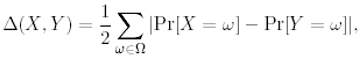• $$\Omega$$为变量X和Y的采样空间
• 统计距离为1，当且仅当X和Y不相容（每个随机事件$$\omega$$，要么X发生，要么Y发生）
• $$0\leq\Delta(X,Y)\leq 1, \Delta(X,X)=0,\Delta(X,Y)=\Delta(Y,X),\Delta(X,Y)+\Delta(Y,Z)\geq \Delta(X,Z)$$
• 三种假设

• Discrete Logarithm DL假设（给$$g^x$$，难求$$x$$
• (Computational) Diffie-Hellman DH假设（给$$g,\ g^x,\ g^y$$，难求$$g^{xy}$$
• Decision Diffie-Hellman DDH假设（知道$$g,\ g^x,\ g^y,\ g^z$$，难以确定是否$$z=xy\ mod\ n$$
• 证明困难程度依次下降
• 随机自规约：一个问题的实例$$I$$满足：

• $$I$$构造出一个或多个随机实例$$I'$$，后者是独立于前者的均匀分布
• 如果得到随机实例的解，则从$$I'$$的解得到$$I$$的答案
• 以上两步要在多项式时间内完成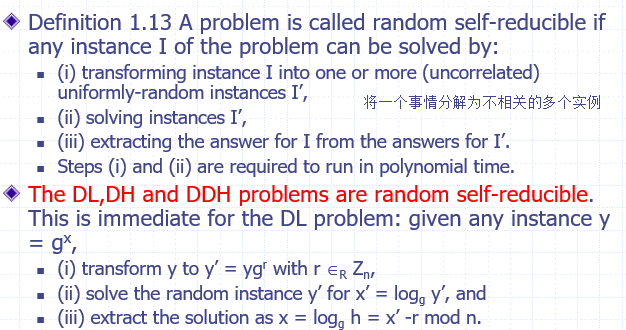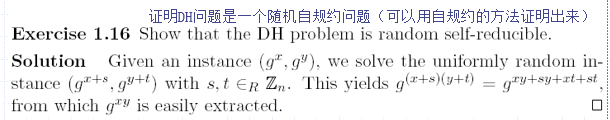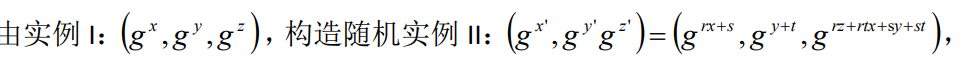• 计算安全的形式：一个方案为$$(t,\epsilon)$$安全，如果每个运行时间最多为t的攻击者最多以$$\epsilon$$的概率攻破——当运行时间和成功概率为安全参数n（双方生成密钥时，以n为安全参数，攻击者也知道）的函数时：

• 多项式时间概率算法：多项式时间内运行的概率算法
• 多项式时间算法：在最坏情况下时间复杂度为$$O(n^k)$$的算法，n为问题规模，k为常数
• 多项式时间算法的基础上，还允许算法获取到随机数（另一种表述为，算法执行中存在一个随机选择下一步的过程，相同输入经过该算法可能会得到不同结果）
• 可忽视函数：（小的成功概率，指比任何关于n的多项式的倒数级别更小）比任何多项式的倒数都增长得慢的函数，称为可忽略的，例如$$2^{-n}$$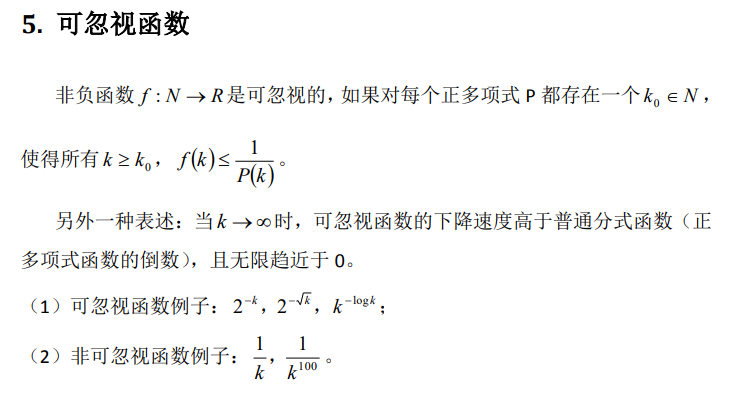• 渐进安全：如果每一个PPT（概率多项式时间）攻击者攻破一个方案的概率是可忽略的，则方案是安全的

### 两方Diffie-Hellman密钥交换

• 协议内容

• 双方约定素数阶有限域$$F_p$$和其乘法循环群一个生成元$$g$$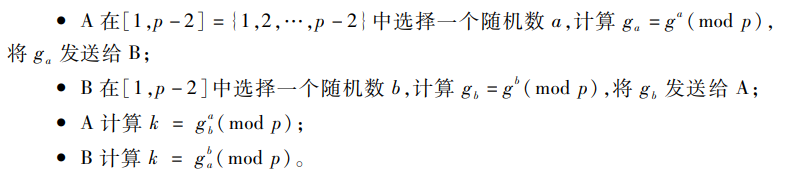• 将协商出的共享秘密值$$k$$用于一个哈希函数的输入，其输出作为会话密钥——因为k是循环群中的元素，不能直接用作对称密钥，并且可能存在较多冗余，哈希函数能够去除这种冗余

• 被动攻击：能够抵御

• 安全性依赖于求解离散对数（CDH）问题的困难性——攻击者截取$$g,g_a,g_b$$，求解$$k=g^{ab}$$
• 必须假设使用的循环群中DDH问题是困难的——攻击者只想知道与$$k$$有关的任何信息
• 中间人攻击：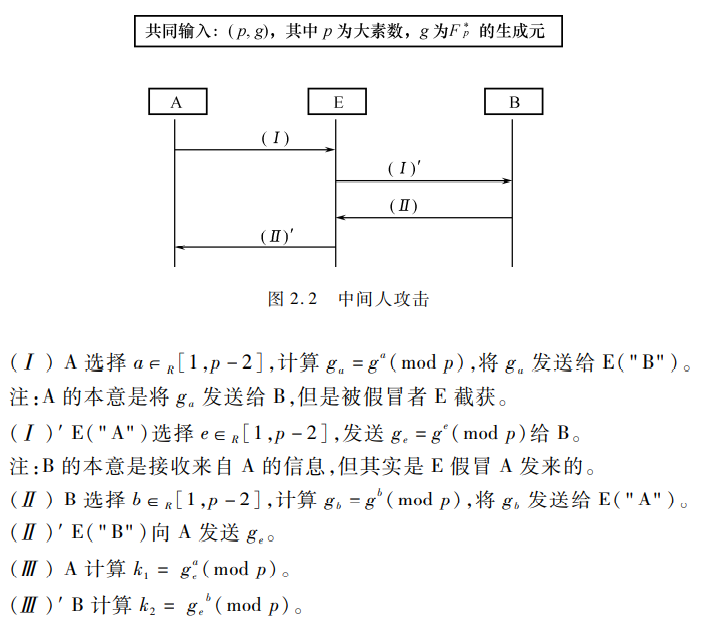• 此时攻击者E就可以计算$$k_1=g_a^e\ mod\ p$$$$k_2=g_b^e\ mod\ p$$
• 不具有密钥的认证功能——协议参与者没有对收到的信息进行认证，以确信消息确实来自认定的通信方
• 端到端协议（station-to-station）：对Diffie-Hellman的改进，假设Torrent为可信中心

• 约定：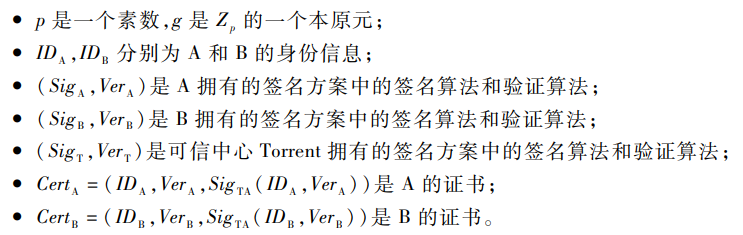• 过程：（使用参与方的数字签名保证信息来源真实性）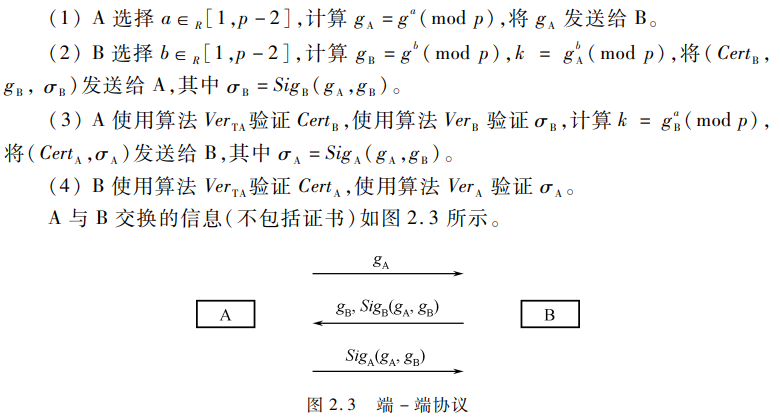• 基于密码加密的协议
• 假设两个参与者共享一个口令
• $$E$$为一个加密算法，以口令和消息作为输入，输出为密文，记为$$C=E_{pw}(m)$$$$D$$为解码算法
• 过程：
• 参与者A选择均匀选择一个$$x_A\in Z_n$$，发送$$E_{pw}(y_A),y_A=g^{x_A}$$给B
• 类似的，参与者B也发送$$E_{pw}(y_B)$$给A
• 则共享密钥为$$k=g^{x_Ax_B}$$
• 被动攻击者只能看到加密后的内容，不会泄露pw的信息，而且$$x_A$$服从均匀分布，也是未知的
• 主动攻击者需要猜出pw，才能实现中间人攻击